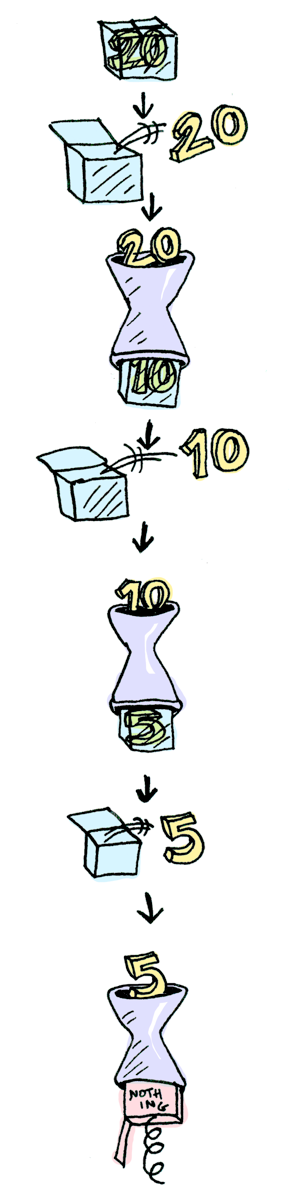### 前言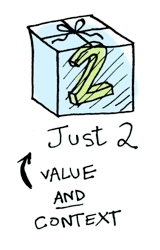## Functors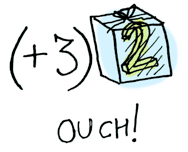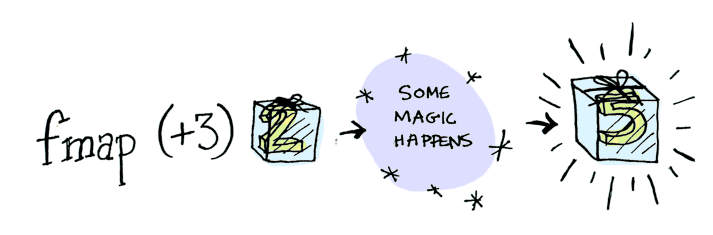fmap怎么知道该如何解析`Just`？换一个其他像`Only`之类的类型，还能解析吗？所以就需要Functor（函子）来完成`定义`的的操作。

Functor是一种数据类型：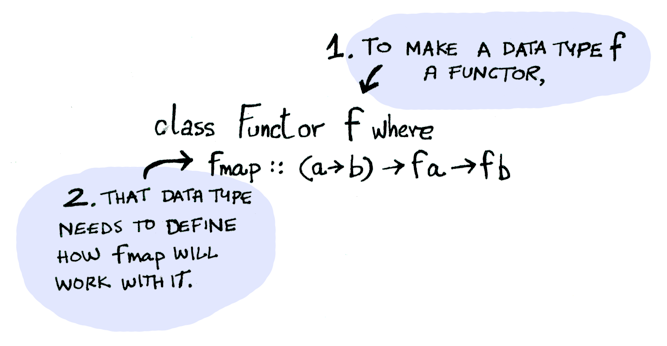Functor定义了fmap的行为：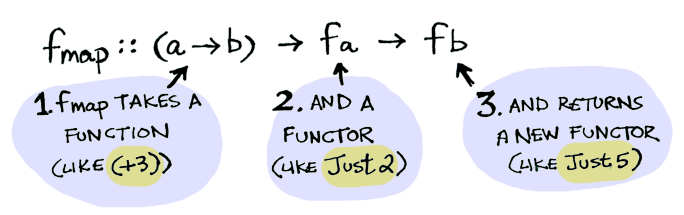fmap有两个入参和一个出参，入参分别是一个函数和一个带盒子的值，出参是一个带盒子的值，可以这样使用：

``````fmap (+3) (Just 2)
-- Just 5``````

``````instance Functor Maybe where
fmap func (Just val) = Just (func val)
fmap func Nothing = Nothing``````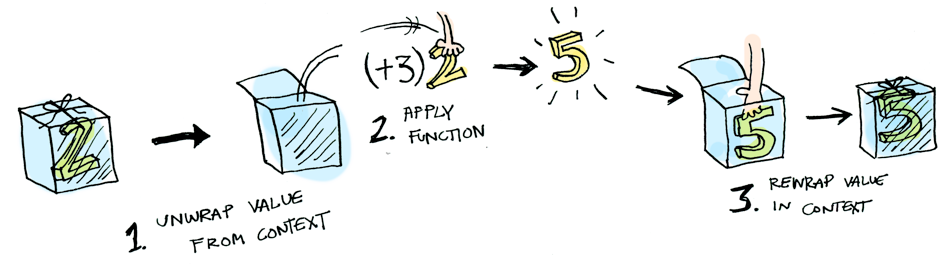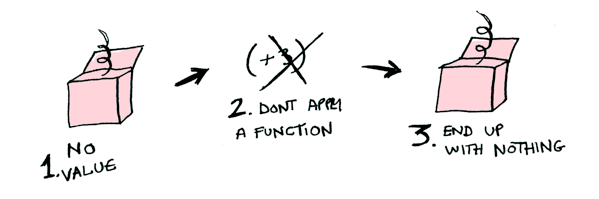``````fmap (+3) Nothing
-- Nothing``````

``````Response res = Request.get(url);
if (res.get("data") != null) {
return res.data;
} else {
return null;
}``````

``fmap (get("data")) (Response res)``

Haskell提供了fmap函数的语法糖`<\$>`简化fmap的写法：

``getData <\$> (Response res)````````instance Functor [] where
fmap = map``````

### Applicatives

`Applicatives`是另一个概念，我们之前说数据被放在盒子里，如果函数也被放在盒子里呢？Haskell的系统提供了操作符`<*>`用于处理盒子里的函数：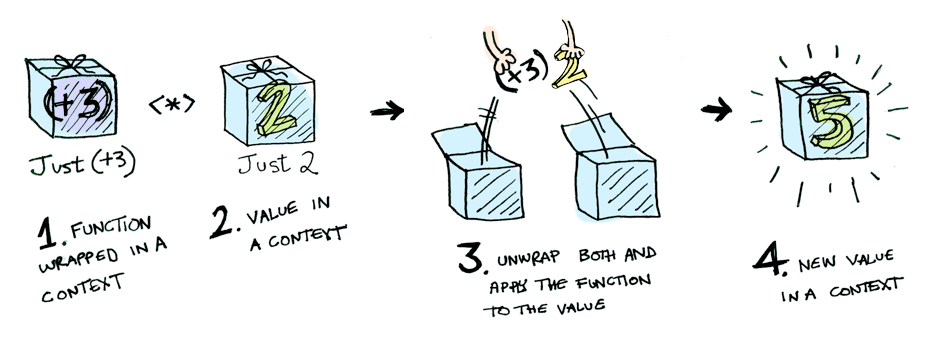``Just (+3) <*> Just 2 == Just 5``

``````[(*2), (+3)] <*> [1, 2, 3]
-- [2, 4, 6, 4, 5, 6]``````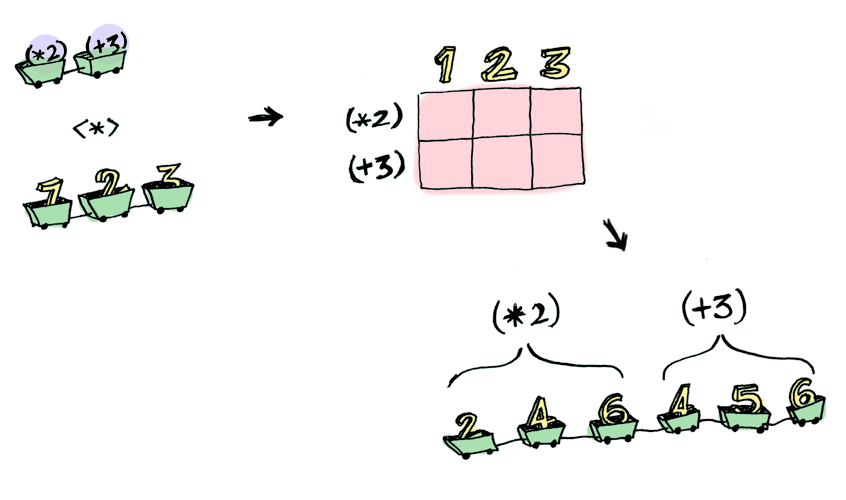``````half x = if even x
then Just (x `div` 2)
else Nothing``````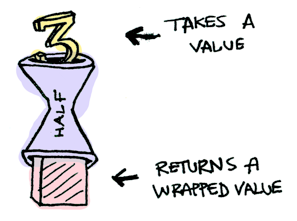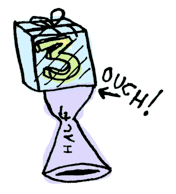`>>=`可以解决这个问题：

``````Just 3 >>= half
-- Nothing``````

`>>=`操作符把`Just 3`变成了`3`放在`half`中进行计算。`Monad`是一个数据类型，定义了`>>=`的行为：

``````class Monad m where
(>>=) :: m a -> (a -> m b) -> m b``````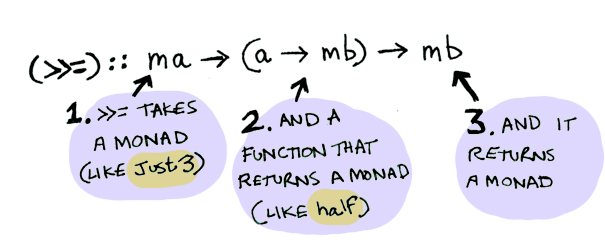``````instance Monad Maybe where
Nothing >>= func = Nothing
Just val >>= func = func val``````

`>>=`还支持链式的操作：

``````Just 20 >>= half >>= half >>= half
-- Nothing``````